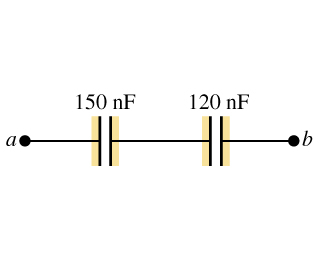# Problem: For the capacitor network shown in (Figure 1) , the potential difference across ab is 48 V .A. Find the charge on the 150 nFcapacitor.B. Find the charge on the 120 nFcapacitor.C. Find the total energy stored in the network.D. Find the energy stored in the 150nF capacitor.E. Find the energy stored in the 120nF capacitor.F. Find the potential difference across the 150 nF capacitor.G. Find the potential difference across the 120 nF capacitor.

###### FREE Expert Solution

For 2 capacitors in series, the equivalent capacitance is:

$\overline{){{\mathbf{C}}}_{\mathbf{e}\mathbf{q}}{\mathbf{=}}\frac{{\mathbf{C}}_{\mathbf{1}}{\mathbf{C}}_{\mathbf{2}}}{{\mathbf{C}}_{\mathbf{1}}\mathbf{+}{\mathbf{C}}_{\mathbf{2}}}}$

The charge stored by a capacitor:

$\overline{){\mathbf{Q}}{\mathbf{=}}{\mathbf{C}}{\mathbf{V}}}$

Energy stored in a capacitor:

$\overline{){\mathbf{U}}{\mathbf{=}}\frac{{\mathbf{Q}}^{\mathbf{2}}}{\mathbf{2}\mathbf{C}}}$

Equivalent capacitance:

Ceq = (150)(120)/(150 + 120) = 66.67 nF

A.

Charge, Q on 150 nF capacitor:

Q = (66.67 × 10-9)(48) = 3.2 × 10-6 C

The charge on the 150 nF capacitor is 3.2 × 10-6 C.

86% (312 ratings)###### Problem Details

For the capacitor network shown in (Figure 1) , the potential difference across ab is 48 V .A. Find the charge on the 150 nFcapacitor.

B. Find the charge on the 120 nFcapacitor.

C. Find the total energy stored in the network.

D. Find the energy stored in the 150nF capacitor.

E. Find the energy stored in the 120nF capacitor.

F. Find the potential difference across the 150 nF capacitor.

G. Find the potential difference across the 120 nF capacitor.